﻿ 求解基于lp+l2范数问题的共轭梯度法 Conjugate Gradient Method for lp+l2 Norm Problems

Vol. 08  No. 03 ( 2019 ), Article ID: 29317 , 12 pages
10.12677/AAM.2019.83057

Conjugate Gradient Method for ${l}_{p}+{l}_{2}$ Norm Problems

Jiaming Zhan, Caiying Wu*

School of Mathematical Sciences, Inner Mongolia University, Hohhot Inner Mongolia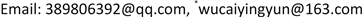Received: Feb. 28th, 2019; accepted: Mar. 13th, 2019; published: Mar. 20th, 2019ABSTRACT

A new model is proposed for sparse signal reconstruction. We smooth our model by smoothing absolute value function. Then we use a new tri-term conjugate gradient method to restore sparse signal. The effect of different parameters on sparse signal recovery is tested. The numerical results show that our algorithm is efficient.

Keywords:Sparse Signal Recovery, Conjugate Gradient Method, ${l}_{p}+{l}_{2}$ Norm, Global Convergence1. 引言

$\underset{x\in {R}^{n}}{\mathrm{min}}{‖x‖}_{0},\text{s}\text{.t}.Ax=b.$ (1)

$\underset{x\in {R}^{n}}{\mathrm{min}}{‖x‖}_{1},\text{s}\text{.t}.Ax=b.$ (2)

(2)称作1范数模型，在适当的假设下，由文献  的定理1.3可知，模型(2)可以较精确地恢复原始信号。

$\underset{x\in {R}^{n}}{\mathrm{min}}{‖x‖}_{\text{p}},\text{s}\text{.t}.Ax=b.$ (3)

2. 模型及其性质

$\underset{x\in {R}^{n}}{\mathrm{min}}{\lambda }_{1}{‖x‖}_{p}+{\lambda }_{2}{‖x‖}_{2},\text{s}\text{.t}.Ax=b.$

$\underset{x\in {R}^{n}}{\mathrm{min}}{\lambda }_{1}{‖x‖}_{p}+{\lambda }_{2}{‖x‖}_{2}+\frac{1}{2}{‖Ax-b‖}_{2}^{2}.$ (4)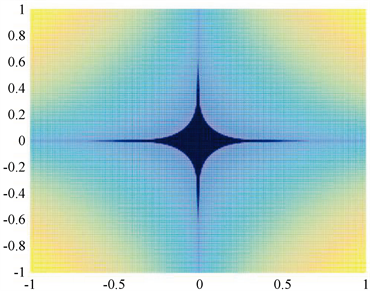Figure 1. R = 0 norm imageFigure 2. R = 1 norm image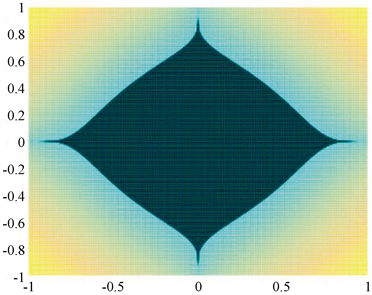Figure 3. R = 10 norm image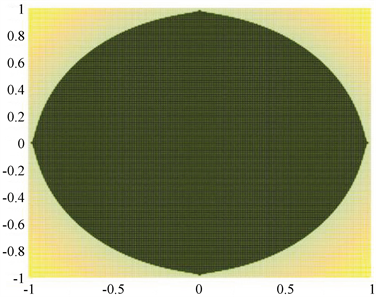Figure 4. R = 100 norm image

${\phi }_{\text{1}}\left(\epsilon ,t\right)=\left\{\begin{array}{l}t,t\ge \frac{\epsilon }{2},\\ \frac{{t}^{2}}{\epsilon }+\frac{\epsilon }{4},-\frac{\epsilon }{2}${\phi }_{\text{2}}\left(\epsilon ,t\right)=\left\{\begin{array}{l}\frac{{t}^{2}}{2\epsilon },|t|\le \epsilon ,\\ |t|-\frac{\epsilon }{2},|t|>\epsilon .\end{array}$ (5)

$\mathrm{min}{\lambda }_{1}{\varphi }_{i}\left(\epsilon ,x\right)+{\lambda }_{2}{‖x‖}_{2,\mu }+\frac{1}{2}{‖Ax-b‖}_{2}^{2}.$ (6)

${\varphi }_{i}\left(x\right)={\left[\underset{j=1}{\overset{n}{\sum }}{\phi }_{i}{\left(\epsilon ,{x}_{j}\right)}^{p}\right]}^{\frac{1}{p}},i=1,2,$ (7)

${‖x‖}_{2,\mu }=\sqrt{{x}_{1}^{2}+{x}_{2}^{2}+\cdots +{x}_{n}^{2}+{\mu }^{2}},\mu >0.$

${G}_{i}\left(x\right)={\lambda }_{1}{\varphi }_{i}\left(\epsilon ,x\right)+{\lambda }_{2}{‖x‖}_{2,\mu }+\frac{1}{2}{‖Ax-b‖}_{2}^{2},\left(i=1,2\right).$ (8)

$\nabla {G}_{i}\left(x\right)={\lambda }_{1}\nabla {\varphi }_{i}\left(\epsilon ,x\right)+{\lambda }_{2}\nabla {‖x‖}_{2,\mu }+{A}^{\text{T}}\left(Ax-b\right).$ (9)

$‖h\left(x\right)-h\left(y\right)‖\le L‖x-y‖,\forall x,y\in D.$

$|h\left(x\right)-h\left(y\right)|=|\nabla {h}^{\text{T}}\left(\xi \right)\left(x-y\right)|\le ‖\nabla h\left(\xi \right)‖\cdot ‖x-y‖$ ，其中 $\xi$ 位于 $x,y$ 之间。

$|f\left(x\right)|\le M,|g\left(x\right)|\le M,|f\left(x\right)-f\left(y\right)|\le {L}_{1}‖x-y‖,|g\left(x\right)-g\left(y\right)|\le {L}_{2}‖x-y‖,\forall x,y\in D.$

$\forall x,y\in D$ ，有：

$\begin{array}{l}|f\left(x\right)g\left(x\right)-f\left(y\right)g\left(y\right)|\\ =|f\left(x\right)g\left(x\right)-f\left(y\right)g\left(x\right)+f\left(y\right)g\left(x\right)-f\left(y\right)g\left(y\right)|\\ \le |g\left(x\right)||f\left(x\right)-f\left(y\right)|+|f\left(y\right)||g\left(x\right)-g\left(y\right)|\\ \le M{L}_{1}‖x-y‖+M{L}_{2}‖x-y‖\\ =M\left({L}_{1}+{L}_{2}\right)‖x-y‖.\end{array}$

${{\phi }^{\prime }}_{i}\left(\epsilon ,t\right)=\left\{\begin{array}{l}\mathrm{sgn}\left(t\right),|t|\ge \frac{\epsilon }{2},\\ \frac{2t}{\epsilon },|t|<\frac{\epsilon }{2}.\end{array}$

$\frac{\epsilon }{2}>{t}_{1}>{t}_{2}>-\frac{\epsilon }{2}$ 时，有：

$|{{\phi }^{\prime }}_{1}\left(\epsilon ,{t}_{1}\right)-{{\phi }^{\prime }}_{1}\left(\epsilon ,{t}_{2}\right)|\le |\frac{2{t}_{1}}{\epsilon }-\frac{2{t}_{2}}{\epsilon }|=\frac{2}{\epsilon }|{t}_{1}-{t}_{2}|.$

${t}_{1}>\frac{\epsilon }{2}>{t}_{2}>-\frac{\epsilon }{2}$ 时，有：

$|{{\phi }^{\prime }}_{1}\left(\epsilon ,{t}_{1}\right)-{{\phi }^{\prime }}_{1}\left(\epsilon ,{t}_{2}\right)|=|1-\frac{2{t}_{2}}{\epsilon }|<|\frac{2{t}_{1}}{\epsilon }-\frac{2{t}_{2}}{\epsilon }|=\frac{2}{\epsilon }|{t}_{1}-{t}_{2}|.$

$\frac{\epsilon }{2}>{t}_{1}>-\frac{\epsilon }{2}>{t}_{2}$ 时，有：

$|{{\phi }^{\prime }}_{1}\left(\epsilon ,{t}_{1}\right)-{{\phi }^{\prime }}_{1}\left(\epsilon ,{t}_{2}\right)|=|\frac{2{t}_{1}}{\epsilon }+1|<|\frac{2{t}_{1}}{\epsilon }-\frac{2{t}_{2}}{\epsilon }|=\frac{2}{\epsilon }|{t}_{1}-{t}_{2}|.$

${t}_{1}>\frac{\epsilon }{2}>-\frac{\epsilon }{2}>{t}_{2}$ 时，有：

$|{{\phi }^{\prime }}_{1}\left(\epsilon ,{t}_{1}\right)-{{\phi }^{\prime }}_{1}\left(\epsilon ,{t}_{2}\right)|=2=\frac{2}{\epsilon }\epsilon \le \frac{2}{\epsilon }|{t}_{1}-{t}_{2}|.$

${t}_{1}>{t}_{2}>\frac{\epsilon }{2}$$-\frac{\epsilon }{2}>{t}_{1}>{t}_{2}$ 时，有：

$|{{\phi }^{\prime }}_{1}\left(\epsilon ,{t}_{1}\right)-{{\phi }^{\prime }}_{1}\left(\epsilon ,{t}_{2}\right)|=0\le \frac{2}{\epsilon }|{t}_{1}-{t}_{2}|.$

${L}_{1}=\frac{2}{\epsilon }$ ，同理可证存在常数 ${L}_{2}$ 使得 ${{\phi }^{\prime }}_{2}\left(t\right)$ 在紧集D上Lipschitz连续。

$|{{\phi }^{\prime }}_{i}\left(\epsilon ,{t}_{1}\right)-{{\phi }^{\prime }}_{i}\left(\epsilon ,{t}_{2}\right)|\le {L}_{m}|{t}_{1}-{t}_{2}|,\forall {t}_{1},{t}_{2}\in D,i=1,2.$

${\left[\nabla {\varphi }_{i}\left(\epsilon ,x\right)\right]}_{j}={\left(\underset{j=1}{\overset{n}{\sum }}{\phi }_{i}^{p}\left(\epsilon ,{x}_{j}\right)\right)}^{\frac{1-p}{p}}{\phi }_{i}^{p-1}\left(\epsilon ,{x}_{j}\right){\phi }^{\prime }\left(\epsilon ,{x}_{j}\right).$

${\phi }_{i}$ 连续可微，且 $\epsilon \ne 0,{\phi }_{i}\ne 0$ ，我们有 ${\left(\underset{j=1}{\overset{n}{\sum }}{\phi }_{i}^{p}\left(\epsilon ,{x}_{j}\right)\right)}^{\frac{1-p}{p}}$${\phi }_{i}^{p-1}$ 均连续可微。假设集合D有界，则D为紧集，由引理1知 ${\left(\underset{j=1}{\overset{n}{\sum }}{\phi }_{i}^{p}\left(\epsilon ,{x}_{j}\right)\right)}^{\frac{1-p}{p}}$${\phi }_{i}^{p-1}$ 在紧集D上Lipschitz连续。

${\left(\underset{j=1}{\overset{n}{\sum }}{\phi }_{i}^{p}\left(\epsilon ,{x}_{j}\right)\right)}^{\frac{1-p}{p}}\cdot {\phi }_{i}^{p-1}\left(\epsilon ,{x}_{j}\right)\cdot {\phi }^{\prime }\left(\epsilon ,{x}_{j}\right)$ 在紧集D上Lipschitz连续，记Lipschitz常数为 ${L}_{D}$ ，那么有：

$‖\nabla {\varphi }_{i}\left(\epsilon ,x\right)-\nabla {\varphi }_{i}\left(\epsilon ,y\right)‖\le \underset{j=1}{\overset{n}{\sum }}‖{\left[\nabla {\varphi }_{i}\left(\epsilon ,x\right)\right]}_{j}-{\left[\nabla {\varphi }_{i}\left(\epsilon ,y\right)\right]}_{j}‖\le n{L}_{D}‖x-y‖.$

$\nabla {\varphi }_{i}\left(\epsilon ,x\right)$ 在紧集D上Lipschitz连续。

$‖\nabla {‖x‖}_{2}-\nabla {‖y‖}_{2}‖\le n{L}_{c}‖x-y‖.$

$\begin{array}{l}‖\nabla {G}_{i}\left(x\right)-\nabla {G}_{i}\left(y\right)‖\\ =‖{\lambda }_{1}\nabla {\varphi }_{i}\left(\epsilon ,x\right)+{\lambda }_{2}\nabla {‖x‖}_{2,\mu }+{A}^{\text{T}}\left(Ax-b\right)-{\lambda }_{1}\nabla {\varphi }_{i}\left(\epsilon ,y\right)-{\lambda }_{2}\nabla {‖y‖}_{2,\mu }-{A}^{\text{T}}\left(Ay-b\right)‖\\ \le {\lambda }_{1}n{L}_{D}‖x-y‖+{\lambda }_{2}n{L}_{C}‖x-y‖+{‖A‖}_{2}^{2}‖x-y‖\\ =\left({\lambda }_{1}n{L}_{D}+{\lambda }_{2}n{L}_{C}+{‖A‖}_{2}^{2}\right)‖x-y‖.\end{array}$

3. 基于非精确线搜索的三项共轭梯度法

$\begin{array}{l}{x}_{k+1}={x}_{k}+{\alpha }_{k}{d}_{k},\\ {d}_{k}=\left\{\begin{array}{l}-{g}_{k},k=0,\\ -{g}_{k}+{\beta }_{k-1}{d}_{k-1},k>0.\end{array}\end{array}$

${d}_{k}=\left\{\begin{array}{l}-{g}_{k},k=0,\\ -{g}_{k}+{\beta }_{k-1}{d}_{k-1}-{\theta }_{k-1}{y}_{k-1},k>0.\end{array}$

${d}_{k}=\left\{\begin{array}{l}-{g}_{k},k=0,\\ -{g}_{k}+{\beta }_{k-1}{d}_{k-1}-{\theta }_{k-1}{y}_{k-1},k>0.\end{array}$

${y}_{k}={g}_{k}-{g}_{k-1},{\beta }_{k}=\frac{{g}_{k}^{\text{T}}{y}_{k-1}}{-\eta {g}_{k-1}^{\text{T}}{d}_{k-1}+\mu |{g}_{k}^{\text{T}}{y}_{k-1}|},{\theta }_{k}=\frac{{g}_{k}^{\text{T}}{d}_{k-1}}{-\eta {g}_{k-1}^{\text{T}}{d}_{k-1}+\mu |{g}_{k}^{\text{T}}{y}_{k-1}|}.$

$G\left({x}_{k}+{\alpha }_{k}{d}_{k}\right)>G\left({x}_{k}\right)-2\sigma \left(1-\gamma {\mu }_{0}\right){\alpha }_{k}G\left({x}_{k}\right).$

$\underset{k\to 0}{\text{lim}}‖\nabla G\left({x}_{k}\right)‖=0.$

4. 数值实验

$\mu =1e-5,\epsilon =1e-5,\eta =1,\delta =2,\lambda =1e-6\ast {‖{A}^{\text{T}}b‖}_{\infty },\rho =0.5,\sigma =0.002,\gamma =0.2.$

1) 我们先对绝对值近似函数 ${\phi }_{1}$ 的信号恢复问题进行数值实验，结果如表1表6所示：Table 1. Signal dimension n = 2048, no noise interferenceTable 2. Signal dimension n = 2048, Gauss noise interferenceTable 3. Signal dimension n = 4096, no noise interferenceTable 4. Signal dimension n = 4096, Gauss noise interferenceTable 5. Signal dimension n = 8192, no noise interferenceTable 6. Signal dimension n = 8192, Gauss noise interference

2) 我们再对绝对值近似函数 ${\phi }_{2}$ 的信号恢复问题进行数值实验，结果如表7表12所示：Table 7. Signal dimension n = 2048, no noise interferenceTable 8. Signal dimension n = 2048, Gauss noise interferenceTable 9. Signal dimension n = 4096, no noise interferenceTable 10. Signal dimension n = 4096, Gauss noise interferenceTable 11. Signal dimension n = 8192, no noise interferenceTable 12. Signal dimension n = 8192, Gauss noise interference

5. 主要结论

1) 相对于p范数模型， ${l}_{p}+{l}_{2}$ 范数模型具有更好的恢复效果，二者在时间和迭代步数相近的情况下， ${l}_{p}+{l}_{2}$ 范数模型对初始信号的精度更高。

2) 做 ${\lambda }_{\text{2}}/{\lambda }_{\text{1}}$ 的值对信号恢复效果影响的横向对比， ${\lambda }_{\text{2}}/{\lambda }_{\text{1}}$ 的取值对信号恢复的时间和迭代步数影响并不明显；但是 ${\lambda }_{\text{2}}/{\lambda }_{\text{1}}=1$${\lambda }_{\text{2}}/{\lambda }_{\text{1}}=10$ 时信号的恢复具有更高精度，这是由于当 ${\lambda }_{\text{2}}/{\lambda }_{\text{1}}$ 取值为1~10时2范

3) 做p的值对信号恢复效果影响的纵向对比，显然范数p = 0.6时信号恢复的精度最高，p = 0.3时精度最低。

$\begin{array}{l}\underset{x\in {R}^{n}}{\mathrm{min}}\lambda {‖x‖}_{1}-\tau {‖x‖}_{2},\\ \text{s}\text{.t}\text{.}Ax=b.\end{array}$

2) 文献  中的光滑化绝对值函数是文献  中 ${\phi }_{3}$

3) 文献  中的模型为：

$\underset{x\in {R}^{n}}{\mathrm{min}}{\lambda }_{1}{‖x‖}_{p}^{p}+{\lambda }_{2}{‖x‖}_{2}^{2}+\frac{1}{2}{‖Ax-b‖}_{2}^{2}.$

${\lambda }_{1}{‖x‖}_{p}^{2}+{\lambda }_{2}{‖x‖}_{2}^{2}$${\lambda }_{1}{‖x‖}_{p}+{\lambda }_{2}{‖x‖}_{2}$ 不易产生稀疏解。取p = 0.3，R = 100对比图形如图5图6所示：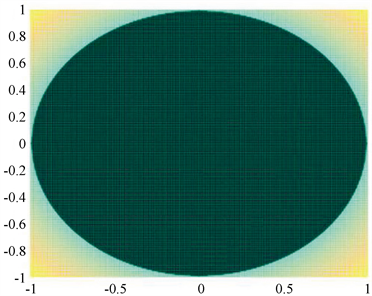Figure 5. ${\lambda }_{1}{‖x‖}_{p}^{p}+{\lambda }_{2}{‖x‖}_{2}^{2}$ norm image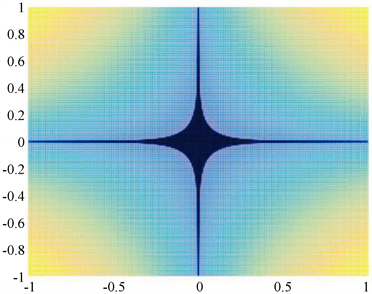Figure 6. ${\lambda }_{1}{‖x‖}_{p}+{\lambda }_{2}{‖x‖}_{2}$ norm image

Conjugate Gradient Method for lp+l2 Norm Problems[J]. 应用数学进展, 2019, 08(03): 512-523. https://doi.org/10.12677/AAM.2019.83057

1. 1. Candes, E.J., Romberg, J. and Tao, T. (2006) Robust Uncertainty Principles: Exact Signal Reconstruction from Highly Incomplete Frequency Information. IEEE Transactions on Information Theory, 52, 489-509. https://doi.org/10.1109/TIT.2005.862083

2. 2. Ge, D., Jiang, X. and Ye, Y. (2011) A Note on the Complexity of Lp Minimization. Mathematical Programming, 129, 285-299. https://doi.org/10.1007/s10107-011-0470-2

3. 3. Candes, E.J. and Tao, T. (2004) Near Optimal Signal Recovery from Random Projections: Universal Encoding Strategies. IEEE Transactions on Information Theory, 52, 5406-5425. https://doi.org/10.1109/TIT.2006.885507

4. 4. Chen, S.S., Donoho, D.L. and Saunders, M.A. (1998) Atomic De-composition by Basis Pursuit. SIAM Journal on Scientific Computing, 20, 33-61. https://doi.org/10.1137/S1064827596304010

5. 5. Daubechies, I., Defries, M. and De Mol, C. (2004) An Iterative Thresholding Algorithm for Linear Inverse Problems with a Sparsity Constraint. Communications on Pure and Applied Mathematics, 57, 1413-1457. https://doi.org/10.1002/cpa.20042

6. 6. Xu, Z.B., Chang, X., Xu, F., et al. (2012) Regularization: A Thresholding Representation Theory and a Fast Solver. IEEE Transactions on Neural Networks and Learning Systems, 23, 1013-1027. https://doi.org/10.1109/TNNLS.2012.2197412

7. 7. Jeevan, K., Pant and Lu, W. (2014) New Improved Algorithms for Compressive Sensing Based on l(p) Norm. Circuits and Systems II: Express Briefs. IEEE Transactions, 61, 198-202.

8. 8. Saheya, B., Yu, C.-H. and Chen, J.-S. (2018) Numerical Comparisons Based on Four Smoothing Func-tions for Absolute Value Equation. Journal of Applied Mathematics and Computing, 56, 131-149. https://doi.org/10.1007/s12190-016-1065-0

9. 9. Bakhtawar, B., Zabidin, S., Ahmad, A., et al. (2017) A New Modified Three-Term Conjugate Gradient Method with Sufficient Descent Property and Its Global Convergence. Journal of Mathematics, 2017, 1-12.

10. 10. Liu, C., Chen, Q., Zhou, B., et al. (2016) L1- and L2-Norm Joint Regularization Based Sparse Signal Reconstruction Scheme. Applied Physics A, 45, 313-323.

11. 11. Zhang, Y. and Ye, W.Z. (2017) Sparse Recovery by the Iteratively Reweighted, Algorithm for Elastic, Minimization. Optimization, 66, 1-11. https://doi.org/10.1080/02331934.2017.1359590

NOTES

*通讯作者。